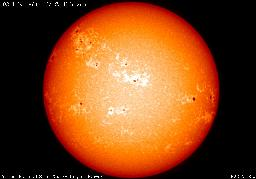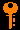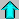# Lecture 11 - Stellar Structure I: Hydrostatic Equilibrium (2/18/99)Radiative Transfer --- | --- Stellar Structure IIReading:
Chapter 16-1, P5-2, P5-6 (ZG4)

Notes:
pages 43 - 46

The solar disk in the 3933A Calcium line.
(From The Sun: A Pictorial Introduction by Charbonneau & White.)

Key Question:## Investigations:

1. A Spherical Star
• What is the mass of a spherical shell of thickness dr at radius r with density rho?
• What is the differential equation of mass continuity?
• What is the mean density of a star given M and R?
• What is the mean density of the Sun? Compared to water?
• What are the important stellar parameters for stellar structure?
• Why should the mass and composition be the controlling variables?
2. Hydrostatic Equilibrium
• What balances gravity in a star?
• What is the pressure gradient needed to support a spherical shell?
• What do we mean by hydrostatic equilibrium?
• What is static about it?
• How do we change independent variables from r to M in the Lagrangian formulation?
• To what other systems does hydrostatic equilibrium apply?
• What is the solution in the atmosphere of the Earth giving the pressure scale height?
3. The Constant Density Star
• What is the solution of P(r) assuming constant density?
• What is the central pressure of a constant density star?
• Why is this a lower limit on the true central pressure of a real star?
• How do we estimate the temperature from the pressure?
• What is the central temperature of a constant density star?
• Is this also a lower limit on the true temperature?
• What is the estimated central pressure and temperature of the Sun?
• What does this imply for an energy source?
4. The Perfect Gas Law
• What is the perfect gas law?
• How is the temperature of a gas related to its rms velocity?
• What is the kinematic explanation for the perfect gas law?
• How do you derive P=nkT for a box of gas?
• Why do we not use the form PV=NRT?
5. Mean Molecular Weight
• What do we mean by a "metal" when we talk about stellar composition?
• What are the mass fractions X, Y, Z for H, He and metals in the Sun?
• What do we mean by mean molecular weight of a gas?
• What is the mean molecular weight of pure neutral hydrogen?
• What is the mean molecular weight of pure ionized hydrogen?
• What is the mean molecular weight of the Sun's interior?
• What is the relation between the kinetic energy and momemtum of a gas particle?
• Since light carries energy, does a photon have momentum even though it is massless?
• What is the relation between pressure, force, and momentum?
• What is the energy density of a bath of radiation at temperature T?
• How do pressure and energy of a gas relate to pressure and energy of radiation?
• Where would you expect radiation pressure to be important in a star?
• Can you use radiation pressure to propel a spacecraft?

## Stellar Structure in Outline:

1. The Pressure-Temperature Thermostat
• Pressure from energy generation in the core balances the gravitational weight of the layers of the star above it.
• When the core is undergoing thermonuclear fusion, the pressure from the energy generation balances gravity.
• Because the rate of nuclear reactions depends very sensitively on the temperature, a slight increase in temperature will cause and increase in the energy generation rate, thus increasing the pressure of the core, thus expanding the core against gravity, thus reducing the temperature (with the decrease in density), thus reducing the energy produced, and the pressure, and radius, and the system comes back to equilibrium.
• This is an example of negative feedback in an astrophysical system. A postive change in one of the parameters causes changes in the system that feed back into a negative change in the original parameter, bringing the system back into an equilibrium. This is like a "cruise control" for the nuclear reactions in the star.
• If the core is not generating thermonuclear energy, like in the original collapse of a protostar, then the gravitational energy of contraction generates heat, and thus pressure against gravity. There is still a negative feedback against temperature increases, though the core will slowly contract to generate the heat to maintain the pressure against the weight of the star.
• Pressure-temperature regulation is only part of the story of stellar structure. We now consider the other factors that go into a stellar model.
2. Stellar Structure
• There are 4 global physical quantities of a star: L=luminosity, T=temperature, M=mass, and R=radius.
• A star can be modeled as a spherically symmetric gas that is in equilibrium, that is it doesn't change with time (at least on timescales short compared to the energy generation timescale).
• We can build a star by calculating the physical properties as a function of radius r within the star.
• There are 2 quantities that depend upon the shells below directly: L(r) the luminosity at any shell generated by the shells below it, and M(r) the mass within the radius r.
• The temperature T(r) is a local quantity, the temperature of the gas in the shell at radius r.
• To help us calculate, we also use 3 other local quantities: the gas mass density rho(r) in kg/m^3 at radius r, the gas pressure p(r), and the energy (luminosity) generation rate E(r).
• We calculate the star model by adding shells consecutively from the center of the star (r=0) to the surface of the star (r=R). At the surface M(R),T(R),L(R) are just the physical quantities we can observer for the star.
• We use four laws to tell us how to make a change in the quantities when we add a shell:
1. Mass - add the mass in the shell to the total
2. Luminosity - add the luminosity generated in the shell to the total
3. Pressure - adjust the pressure to balance the weight of the new shell (reduce the pressure, since the shell contributes to the pressure of lower layers only)
4. Temperature - heat is transported from the hot innner layers to cooler outer layers by convection, radiation or conduction.
• This prescription is carried out through four equations that give the changes in each quantity with radius.
• This purely mathematical model describes the structure of the star. Furthermore, you can take into account the changes with the star's energy production with time, and thus calculate the evolution of the star's structure.Prev Lecture ---Next Lecture ---Astr12 Index ---Astr12 Home

smyers@nrao.edu Steven T. Myers